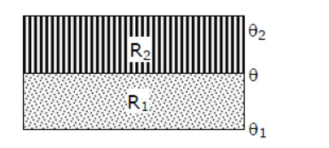# Solve thisQuestion:

The temperature $\theta$ at the junction of two insulating sheets, having thermal resistances $\mathrm{R}_{1}$ and $\mathrm{R}_{2}$ as well as top and bottom temperatures $\theta_{1}$ and $\theta_{2}$ (as shown in figure) is given by :1. $\frac{\theta_{1} R_{2}+\theta_{2} R_{1}}{R_{1}+R_{2}}$

2. $\frac{\theta_{1} R_{2}-\theta_{2} R_{1}}{R_{2}-R_{1}}$

3. $\frac{\theta_{2} R_{2}-\theta_{1} R_{1}}{R_{2}-R_{1}}$

4. $\frac{\theta_{1} R_{1}+\theta_{2} R_{2}}{R_{1}+R_{2}}$

Correct Option: 1

Solution:

(1)

Temperature at the junction is $\theta$.

so using the formula

$\frac{T_{2}-T}{R_{1}}=\frac{T-T_{1}}{R_{2}}$

$\frac{\theta_{2}-\theta}{\mathrm{R}_{2}}=\frac{\theta-\theta_{1}}{\mathrm{R}_{1}}$

$\mathrm{R}_{1}\left(\theta_{2}-\theta\right)=\mathrm{R}_{2}\left(\theta-\theta_{1}\right)$

$\mathrm{R}_{1} \theta_{2}-\mathrm{R}_{1} \theta=\mathrm{R}_{2} \theta-\mathrm{R}_{2} \theta_{1}$

$\mathrm{R}_{1} \theta+\mathrm{R}_{2} \theta=\mathrm{R}_{1} \theta_{2}+\mathrm{R}_{2} \theta_{1}$

$\theta=\frac{R_{1} \theta_{2}+R_{2} \theta_{1}}{R_{1}+R_{2}}$